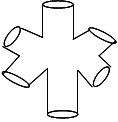## Spaced Out Arrows

The source category in an ordinary topological field theory is always the 1-category n-cob of n dimensional spaces as arrows between (n-1) dimensional spaces as objects. For example, the six punctured spherewith three boundaries labelled in and three boundaries labelled out is considered as an arrow in 2-cob, a category of two dimensional spaces.

In M Theory, on the other hand, we often consider such diagrams to be arrows in a multicategory, since an operad is a multicategory on one object. That is, each little circle boundary element is a separate object in the multicategory, which is hence built up entirely of connected spaces. Allowing for multiple outputs takes us beyond ordinary operads to structures incorporating duals, but starting with the operad case, one might ask for an operad map into a target operad, instead of an ordinary (modular) functor. When the target is based on a vector space, such a map yields an algebra for an operad. Since two dimensional TFTs hinge on the assignment of one (Hilbert) space $V$ to the circle object, it appears there is no loss of information in considering the $\textrm{End}(V)$ operad instead of the entire category of vector spaces.

But 1-categories are not really that interesting. In a 2-category of two dimensional spaces, the one object is a point, and there are two 1-arrows: the arc and the circle (open and closed string). So the Hom 1-category has two objects to map into an algebraic target. In three dimensions the top level has an infinite set of surface objects, labelled by genus and boundary circles. By not allowing arcs, in this case there is one object (the point) and only one 1-arrow (the circle). Such tricategories are better known as braided monoidal categories. If the braiding is to be represented concretely by the knotting of pieces of a three dimensional space, then the product acts on the 2-arrows, namely the surface pieces, so that the simplest $\Sigma_1 \otimes \Sigma_2$ is a concatenation of cylinders and the braiding comes from interacting cylinders which cross over. Arrow composition should be gluing, as usual, but to obtain two compositions (horizontal and vertical) one would now like boundaries marked both in and out and also left and right. This requires surface pieces with at least four circle boundary elements (4 punctured spheres). Such gluings clearly give a well-defined surface, satisfying an interchange law. Such a composition of four surface pieces has a new hole in the centre, contributing to the genus of the composition. Note that this is an attempt to discuss concrete braidings with 2-surfaces bounding 3-spaces, in contrast to the usual situation of knots lying in a fixed 3-space.

## 3 Responses so far »

1. 1### kneemo said,

Kea, what do we get if we replace the circle or its n-dimensional analog with a noncommuative space, such as the finite geometry Connes uses in his model?

2. 2### kneemo said,

Nevermind, I think this just gives us a Lattice TQFT (hep-th/9305080).

3. 3### kneemo said,

Kea, I’m currently reading Isham’s quant-ph/0508225 where he considers the monoid of strings of finite projection operators. This approach essentially looks like Carl’s approach to QFT, using finite products of projection operators.

Before this, Isham considers creating a monoid out of all the self-adjoint operators, but noticed that is not a sub-monoid of monoid of all bounded operators because the product of self-adjoint is not always self-adjoint. A way around this is to define a new product, as is done to create Jordan algebras, so one might be able to follow this path.